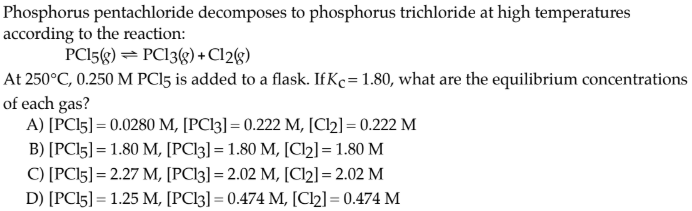# Phosphorus pentachloride decomposes to phosphorus trichloride at high temperatures according to the reaction: PCl5(g) ⇌ PCl3(g) + Cl2(g) At 250°C, 0.250 M PCl5 is added to a flask. If Kc = 1.80, what are the equilibrium concentrations of each gas? A) [PCl5] = 0.0280 M, [PCl3] = 0.222 M, [Cl2] = 0.222 M B) [PCl5] = 1.80 M, [PCl3] = 1.80 M, [Cl2] = 1.80 M C) [PCl5] = 2.27 M, [PCl3] = 2.02 M, [Cl2] = 2.02 M D) [PCl5] = 1.25 M, [PCl3] = 0.474 M, [Cl2] = 0.474 M